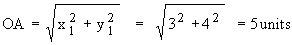Home MonkeyNotes Printable Notes Digital Library Study Guides Study Smart Parents Tips College Planning Test Prep Fun Zone Help / FAQ How to Cite New Title Request

 8.2 Relations, Graphs and symmetry 1) A relation is a set of ordered pairs of numbers. Relations are usually written in the set builder notation. For example {(x, y) | y Ł x}, { (x, y) | y = x2 + 1 } etc. In most cases we just write y Ł x, y = x2 + 1 etc. Many a times we just give the list of ordered pairs { (1,2) , (3,5), (-2,6) } A relation can be best understood by sketching its graph. First, we will study graphic equations on the coordinate plane. To graph an equation on the co-ordinate plane, find the solutions by giving a value to one variable and this will help solve the other variable. Then graph the solutions. Example Find the distance of the point A(3,4) from 0 [origin]. Solution : A (x1, y1) = ( 3, 4 ) Distance of A from the origin 0, isExample Find the distance between the points (2,3) and (7,8) Solution : Let A (x1, y1) = (2, 3) and B (x2 , y2) = (7, 8) Distance between points A and B, is.Example The distance between the point A (8,8) and a point B on the y - axis is 10. Find the Coordinates of B. Solution : Since point B is on the y-axis, B is (0, y2) Let A (x1, y1) = (8, 8) The distance between A and B, isIndex 8.1 Definition8.2 Relations, Graphs and Symmetry8.3 Slopes and Intercepts Chapter 9
 Search: All Products Books Popular Music Classical Music Video DVD Toys & Games Electronics Software Tools & Hardware Outdoor Living Kitchen & Housewares Camera & Photo Cell Phones Keywords: